Quantum Computing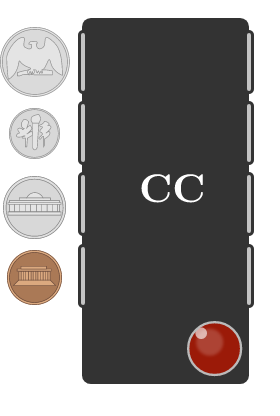This course will teach you how to compute using the principles of quantum mechanics. We’ll start with a simple puzzle and solve it using qubits in two ways:

First, we’re going to limit our qubits to their two computational states: $1$ and $0$.

Next, we’ll try using qubits in a superposition: any combination of two computational states $\ket{1}$ and $\ket{0}$. You will build a real quantum circuit and run it yourself to see the quantum advantage.

Let’s get started.

A Black Box Puzzle

You’re given four coins. These coins look authentic, but you believe that one or more of them may be counterfeit and thus weigh slightly more or less than a real coin.Thankfully, you’re also given a coin checker: a black box with a mechanism very similar to a vending machine which can accept one coin at a time. Put in a real coin, and it will pass through no problem. But put in a counterfeit coin, which weighs a different amount, and it will pass through but also set off a brief alarm, alerting you that the coin you put in was counterfeit.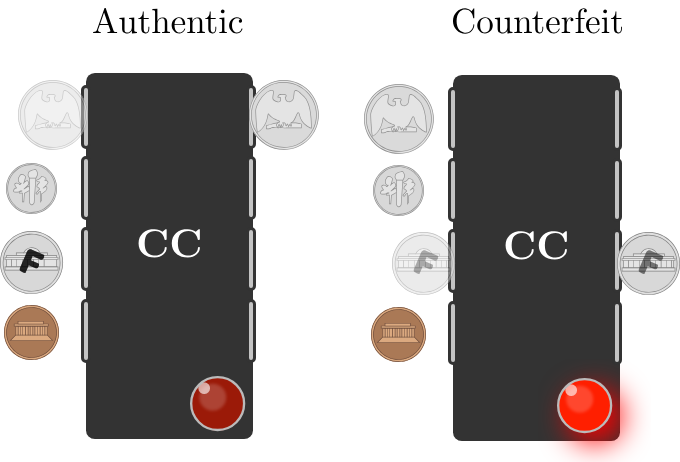How many times will you have to use your coin checker to find which (if any) coins are counterfeit?

A Black Box Puzzle

In an earlier quiz, we said that any problem can be solved using simple logical operations. Let’s try to restate this coin checking problem using qubits.

Here is our coin checker again, except instead of inserting coins, we’re setting states on a circuit. Each row is a qubit, and the first four represent the coins; a $0 \%$ means a coin isn’t inserted, and all qubits begin this way. A $100 \%$ means we’ve inserted that coin. The final row of the coin checker is the alarm. If it is marked $100 \%$, then the coin which was inserted is counterfeit.

We have to use a logical operation to flip the qubit and insert the coin. This simplest of the logical operation is called $\mathbf{NOT}$, but it will be called $\mathbf{X}$ in this course, as is a convention for quantum computing: \begin{aligned} \ket{0} &\ce{->[\mathbf{X}]} \ket{1}\\\\ \ket{1} &\ce{->[\mathbf{X}]} \ket{0}. \end{aligned} Play around with inserting the coins one at a time by dragging in the $\mathbf{X}$ gate. Which coins are counterfeit?

A Black Box Puzzle

For those who prefer to look at this puzzle more mathematically, our coin checker is a black box which passes the input through unchanged, but performs some mathematical function that gets outputted as an alarm.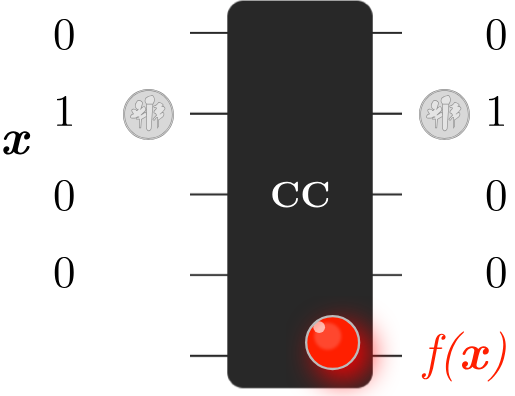The input is some string $x$ with a length of $4$ which describes which coin is being weighed. A $1$ in a position implies that coin is being measured.

The checker somehow “knows” which coins are counterfeit and this knowledge is used to output the function $f(x)$ and set off the alarm.

A Black Box Puzzle

For each $1$ bit we input to the black box checker, we get a bit more information about which coins are counterfeit.

Here’s the logic circuit representation of this puzzle again with four new coins (remember, they're in the first four rows, and you insert them one at a time with the $\mathbf{X}$ operation).

The goal of this puzzle is to identify which coins are counterfeit in as few uses of the checker as possible. After four checks of one coin at a time, how could you represent the information you've gained from the coin checker (i.e. which coins are counterfeit) as a string of four bits?

A Black Box Puzzle

We’ve learned that while bits can only be either $0$ or $1$, a qubit can be a combination of different states at the same time: a superposition. Instead of checking the first bit, then the second, and so on, can we use a superposition to check every bit at once?

This is exactly how many quantum algorithms work: when a qubit in superposition passes through a circuit, the circuit operates on all of the states simultaneously, so it doesn't require separate queries. This approach is so routine that there is a quantum gate called $\mathbf{H}$ designed to prepare this sort of combination: $\ket{0} \ce{->[\mathbf{H}] \ket{0}} + \ket{1}.$ This is the most important quantum gate that you will use in this course, and it is often the first choice to transform a classical algorithm into a quantum one.

While there are $16$ different possible input strings to our checker, using $\mathbf{H}$ on each qubit can prepare a superposition which combines them all.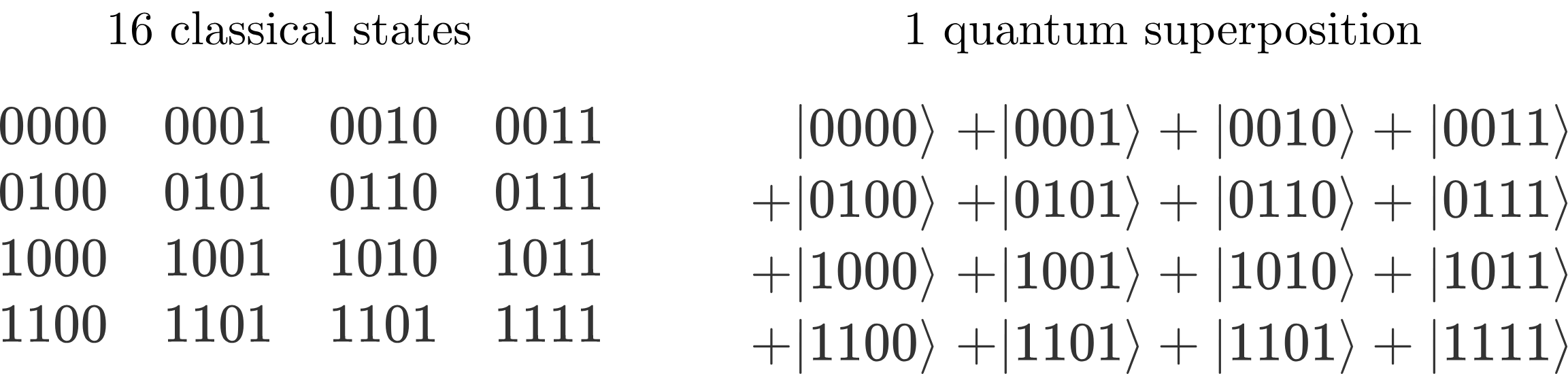A Black Box Puzzle

The new superposition state that we’ve prepared for the checker has produced nothing but a muddled mess.

By putting in a combination of every possible input, we’ve yielded an incomprehensible combination of an alarm and no alarm, along with seemingly no information about which coins are counterfeit.

But all is not lost, the state coming out of the checker has changed. Every combination of inputs containing a single counterfeit coin has been marked with an alarm; we just can't see it yet.

Using a more advanced feature of the simulator above which you'll use in the course, we can read the exact output superposition state: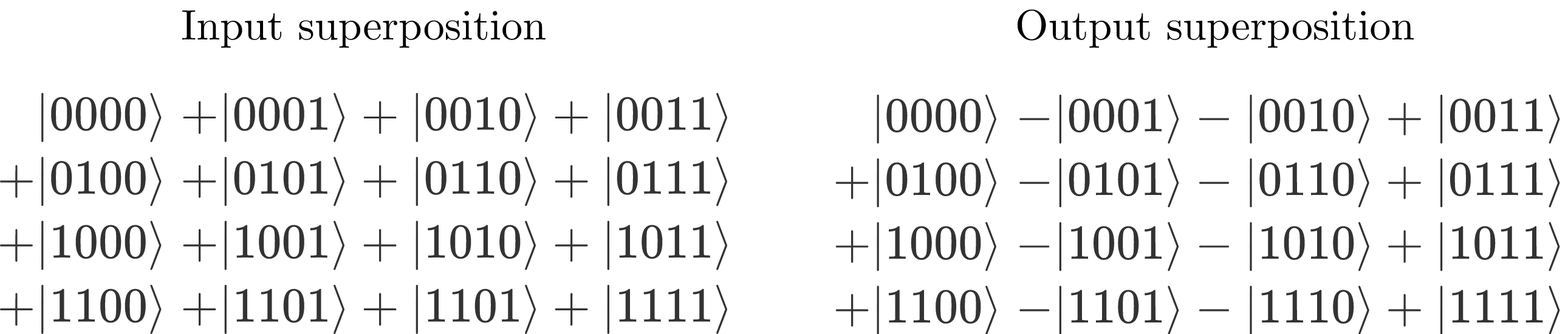How has the state changed? Could we figure out which coins are counterfeit from this state alone?

A Black Box Puzzle

The output superposition of the checker contains all the information we need to see which coins are counterfeit. By comparing some of the input terms to the output ones, we have the following: \begin{aligned} + \ket{1000} & \rightarrow +\ket{1000} \quad \textrm{No alarm}\\ + \ket{0100} & \rightarrow +\ket{0100} \quad \textrm{No alarm}\\ + \ket{0010} & \rightarrow -\ket{0010} \quad \color{#D61F06} \textrm{Alarm!} \color{#333333}\\ + \ket{0001} & \rightarrow -\ket{0001}\quad \color{#D61F06} \textrm{Alarm!} \color{#333333}. \end{aligned} Somehow in only one query, all the possible coin inputs that set off the alarm have been tagged with a $-$ sign.

There's a big problem here, however. As we learned in the last quiz, it's not possible to extract the coefficients of a superposition through measurement—all you'll get is a random chance at any one of these results.

A Black Box Puzzle

The only time that we can be sure of the measurements of a qubit is when it is in only one of the computational states: $\ket{0}$ and $\ket{1}$ will produce deterministic measurements.

Thankfully, the $\mathbf{H}$ operation has another trick up its sleeve: not only does it flip a computational state into a superposition, it can also do the opposite to transform different superpositions back into defined computational states: \begin{aligned} \ket{0} + \ket{1} &\ce{->[\mathbf{H}]} \ket{0} \\ \ket{0} - \ket{1} &\ce{->[\mathbf{H}]} \ket{1}. \end{aligned}

Can you use the $\mathbf{H}$ gate to find the counterfeit coins in a single query?

A Black Box Puzzle

Preparing a superposition state for the oracle, followed by decoding the mixed jumble of a state it yields back into one of the computational states is a common approach in quantum algorithms. In problems like the counterfeit coin puzzle, a brute force search that could take many queries can be sped up significantly. This approach is called quantum parallelism, and we'll become well-versed in the careful choreography of quantum problem-solving in this course.

The construction of this quantum circuit is not meant to be obvious to you now, and we’ll spend much of this course unpacking how superposition and other quantum properties can be leveraged to speed up computation. Throughout the course, drag-and-drop quantum circuits like this one will allow you to try out the computation for yourself, and get a feel for the operations you’re performing as equations never could.

A Black Box Puzzle

×